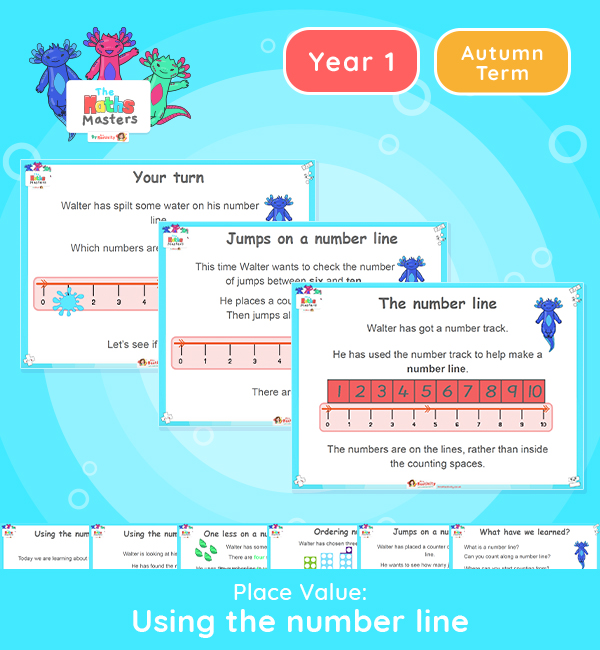# Year 1 | Using the Number Line Lesson Presentation## Year 1 place value resources

This lesson introduce the number line to 10 and how to use it to compare numbers.

Aligned with the maths mastery approach, this Year 1 | Using the Number Line Lesson Presentation is fully editable, and is designed for the Year 1 maths curriculum covering the following maths objectives for the autumn term:

Small Steps: The number line.

NC Links: Count to ten forwards, beginning with 0, or 1, or any given number.. Count, read and write numbers to 10 in numerals and words. Identify and represent numbers using objects and pictorial representations including the number line..

TAF Statements: Working Towards – Read and write numbers in numerals (to 10).

Working At –  Read scales in divisions (of ones)

Greater Depth – Read scales where not all the numbers on the scale are given.

Use reasoning about numbers and relationships to solve more complex problems and explain their thinking.

Ready-to-progress Criteria: 1NPV-2 Reason about the location of numbers to 20 within the linear number system, including comparing using < > and =

Future Applications: Compare and order numbers

Aligned with the order of teaching of the maths mastery approach, use this to help your children get to grips with each mathematical concept. This lesson presentation also includes varied fluency activities, problem solving, and mathematical discussion questions too.

Explore our other year 1 place value resources.

## Recently Viewed